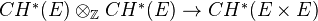# Chow groups

<]]>

<]]>
• ## Online References

Gillet: K-theory and Intersection theory (looks excellent)

Something might be in here.

A very intereting paper by Joshua on motivic DGA.

<]]>
• ## Paper References

Levine in K-theory handbook.

My own notes from Totaro's course, spring 2008.

Seminaire Chevalley: Anneaux de Chow et applications (1958)

Soulé et al: Lectures on Arakelov Geometry. First chapter treats intersection theory for an arbitrary regular noetherian finite-dimensional scheme.

What about Bloch: An elementary presentation... (in Motives vol)?

<]]>

<]]>
• ## Properties

Homotopy invariance and localization. See Levine (page 443). Also Mayer-Vietoris long exact seq. Products. Projective bundle formula.

The Kunneth formula fails for Chow groups, at least over the complex numbers. Counterexample: See Totaro's Algebraic cycles exam, there one shows that the natural homomorphismis not surjective.

<]]>

<]]>

<]]>

<]]>
• ## Computations and Examples

Several papers by Akhtar on K-theory archive.

http://mathoverflow.net/questions/99897/examples-of-chow-rings-of-surfaces mentions surfaces over finite fields

http://mathoverflow.net/questions/11343/algorithm-for-calculating-the-chow-groups-of-a-variety-over-a-finite-field

arXiv:1207.4703 On the Chow groups of certain geometrically rational 5-folds fra arXiv Front: math.NT av Ambrus Pal We give an explicit regular model for the quadric fibration studied in Pirutka (2011). As an application we show that this construction furnishes a counterexample for the integral Tate conjecture in any odd characteristic for some sufficiently large finite field. We study the étale cohomology of this regular model, and as a consequence we derive that these counterexamples are not torsion.

arXiv:1206.2704 On the torsion of Chow groups of Severi-Brauer varieties fra arXiv Front: math.AG av Sanghoon Baek For a large class of central simple algebras we provide upper bounds for the annihilators of the torsion subgroups of the Chow groups of the corresponding Severi-Brauer varieties.

<]]>

<]]>
• ## Some Research Articles

The Chow groups and the motive of the Hilbert scheme of points on a surface, by Mark Andrea de Cataldo and Luca Migliorini: K0415

Saito: On the bijectivity of some cycle maps (in Motives vol)

Pedrini and Weibel: K-theory and Chow groups on singular varieties (1986)

Roberts: Chow's moving lemma. (Appendix to Kleiman's Motives article in the Oslo volume)

Saito et al: We study the higher Chow groups CH^2(X,1) and CH^3(X,2) of smooth, projective algebraic surfaces over a field of char 0.

The oriented Chow ring, by J. Fasel

Chow rings of excellent quadrics, by Nobuaki Yagita: K0787

MR1780429 (2001m:11106) Otsubo, Noriyuki(J-TOKYO) Selmer groups and zero-cycles on the Fermat quartic surface.

Soulé: Groupes de Chow et K-théorie de varietes sur un corps fini (1984)

Biswat and Srinivas: The Chow ring of a singular surface

Kresch: Canonical rational equivalence of intersections of divisors (1999)

Roberts: Recent developments on Serre's multiplicity conjectures: Gabber's proof of the nonnegativity conjecture (1998)

Complex varieties for which the Chow group mod n is not finite (2002)

Laterveer: Algebraic varieties with small Chow groups. Discusses things related to "representability of Chow groups", and the Hodge conjecture.

Green, Griffiths: Formal Deformation of Chow Groups. In Abel volume.

<]]>

<]]>